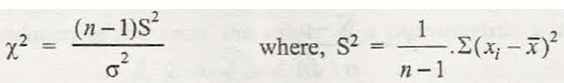A random variable is obtained when a sample is drawn from a population to calculate the sample variance. The results are similar to that of sample mean. With a random sample of n that has a sample variance of S² from a population with a variance σ², then the resultant equation obtained is

X²=   where S²=

Where the variable has a chi square distribution with degrees of freedom, v= n-1 (chi square distribution is where represents the area under the distribution to its rights which is equal to a).If  and  are independent variable random samples of n₁ and n₂, from two normal populations with the same variance, then F=  is another random variable obtained with degrees of freedom v₁= n₁-1 and v₂= -1.

Example:

If two independent random samples of size n₁ = 9 and n₂ = 16 are taken from the normal population, what is the probability that the variance of the first sample will be at least four times as large as that of the second sample ?

Solution:

V₁= 8, v₂= 15

= 4

From F distribution table, F₀.₀₁= 4

Therefore, the probability is 0.01.### Customer Reviews

My Homework Help
Rated 5.0 out of 5 based on 510 customer reviews at